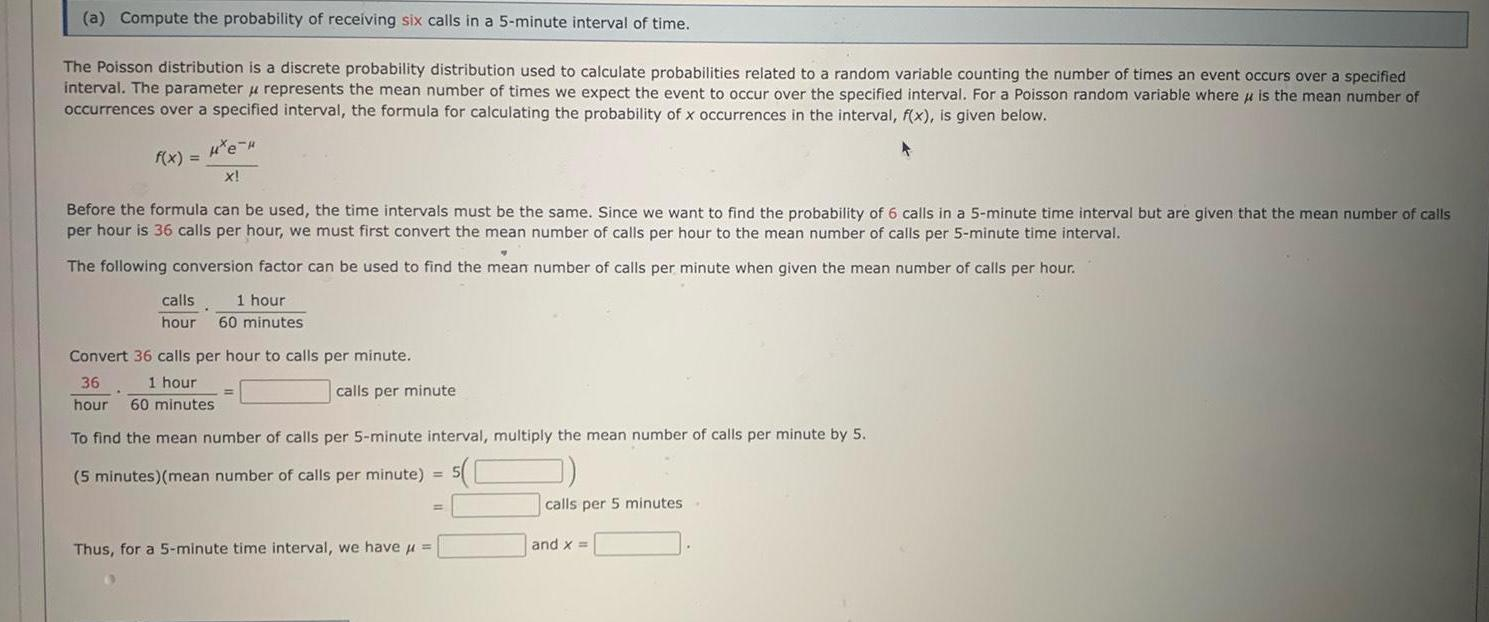Question:

# a Compute the probability of receiving six calls in a 5

Last updated: 11/21/2023a Compute the probability of receiving six calls in a 5 minute interval of time The Poisson distribution is a discrete probability distribution used to calculate probabilities related to a random variable counting the number of times an event occurs over a specified interval The parameter u represents the mean number of times we expect the event to occur over the specified interval For a Poisson random variable where is the mean number of occurrences over a specified interval the formula for calculating the probability of x occurrences in the interval f x is given below He f x X Before the formula can be used the time intervals must be the same Since we want to find the probability of 6 calls in a 5 minute time interval but are given that the mean number of calls per hour is 36 calls per hour we must first convert the mean number of calls per hour to the mean number of calls per 5 minute time interval The following conversion factor can be used to find the mean number of calls per minute when given the mean number of calls per hour calls 1 hour hour 60 minutes Convert 36 calls per hour to calls per minute 36 1 hour hour 60 minutes calls per minute To find the mean number of calls per 5 minute interval multiply the mean number of calls per minute by 5 5 minutes mean number of calls per minute 5 Thus for a 5 minute time interval we have calls per 5 minutes and x